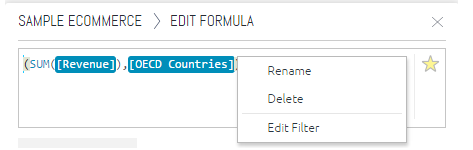# Creating and Editing a Widget Formula

The Data Browser enables you to use formulas (freeform expressions) to determine the values and filters of a widget. A rich variety of functions is provided for your use in formulas.

To create or edit a formula:

1. Open the formula editor in the Data Browser:
• For a new widget, click Select Data, and then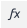.
• For an existing widget, click the edit formula button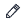.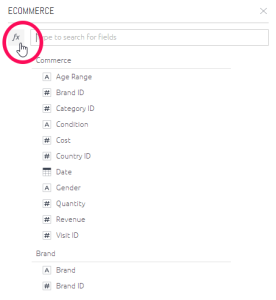The Data Browser then changes to display the Formula Editor, which has the following tabs: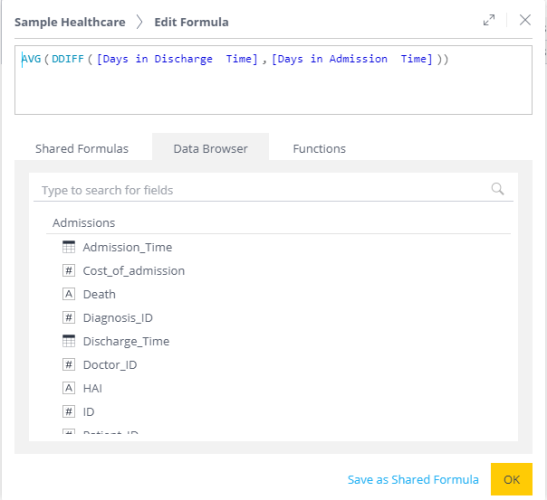* The Shared Formulas tab displays the existing shared formulas that can be used. See Shared Formulas.
* The Data Browser tab provides selectable fields from the data model.
* The Functions tab lists the functions that can be selected for your formula. Hovering over a function in the list displays a tooltip that describes that function.

2. Compose the formula as follows:
• From the Shared Formulas tab, select the relevant shared formula to use, on its own or as part of a larger formula being created.
• From the Data Browser tab, select one or more fields.
• From the Functions tab, select the required functions.
3. Type in the required parts of the formula. To see examples, see Creating Formulas Based on Criteria and Conditions (Filters), and Dashboard Functions Reference.
4. Click OK.

If you hover over a formula and right-click, you have the following options: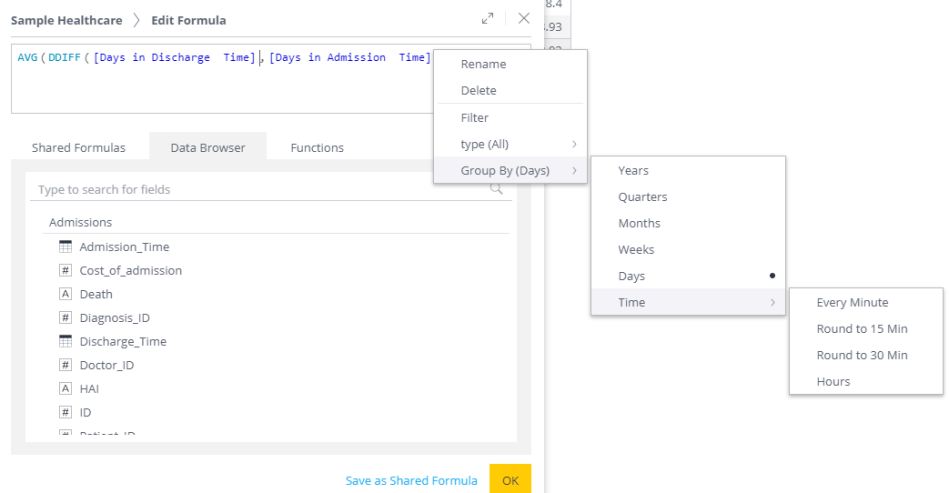• Rename - Renames the formula. For example, give it a name that represents a real-life task or expected result from the formula, or include in the name filters that you have added to the formula.
• Delete - Deletes the formula item.
• Filter - Adds filters to the formula.
• Type - Changes the default aggregation method, for example, from Sum to Average.
• Group By (Days) - Determines the time buckets into which to group the values.

The right-click options for a filtered formula are: